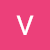# Who defined Division by Zero as Infinity?

The first attempt to define the division by zero was done by Brahmagupta around 628 CE. After that, Bhaskaracarya (c. 1150), while discussing the mathematics of zero in Bijaganita, explains that infinity (ananta-rasi) which results when some number is divided by zero is called khahara. …

# Brāhmasphuṭasiddhānta and the mathematics of Zero

Brāhmasphuṭasiddhānta by Brahmagupta is the first available text that discusses the mathematics of zero.

The following is a verse, that have been taken from the chapter XVIII on algebra

-The sum of positive and negative, if they are equal, is zero.

-The sum of a negative and zero is negative…

# Brahmagupta’s interpolation formula

Brahmagupta was one of the first mathematician to invent and use a second interpolation formula.
The couplet containing the Brahmagupta’s interpolation formula is found in the supplementary part of Khaṇḍakhādyaka, composed around in 665 CE.
An earlier reference is also found in his Dhyana-graha-adhikara.

The couplet in Khandakadyaka, IX,8 :

# Approximation of Sine formula by Bhaskara

Bhaskara (c. 600 — c. 680), one of the remarkable mathematician and an astronomer gave an unique rational approximation of the sine function in his commentary on Aryabhata’s work.

The formula is given in verses 17–19, Chapter VII, Mahabhaskariya

“(Now) I briefly state the rule (for finding the bhujaphala and…

The chapter 12 of Brahmasphutasiddhanta contains information about basic operations such as arithmetic operations, proportions and the “practical mathematics,” such as mixture and series.

This a one such verse from chapter 12 where he discusses about gross area of a triangle and quadrilateral.

This translates to:

“The product of half the sides and countersides is the gross area of a triangle and a quadrilateral. Half the sum of the sides set down four times, and severally lessened by the sides, being multiplied together, the square root of the product is the exact area.”

If we have a quadrilateral with a,b,c,d

# Arithmetic Progression in Brahmasphutasiddhanta

In chapter 12 of his book Brahmasphutasiddhanta, Brahmagupta deals with Arithmetic which also includes geometry.
In verse 17 of the section 3, he deals with arithmetic progression and states:

which translates to:

“The period less one, multiplied by the common difference, being
added to the first term, is the amount of the last. Half the sum of
last and first terms is the mean amount which multiplied by the
period, is the sum of whole.”

# HeapSort using Array

`#include <stdio.h>#define MAX 100void heap_sorting(int elements[], int limit);void show(int elements[], int limit);void restoring(int elements[], int count, int limit);int delete_root(int elements[], int *limit);void heap_builder(int elements[], int limit);int main(){int count;int elements[MAX], limit;printf(“\nEnter Total Number of Elements:\t”);scanf(“%d”, &limit);for(count = 1; count <= limit; count++){printf(“Element No.%d:\t”, count);scanf(“%d”, &elements[count]);}printf(“\nElements To Sort\n”);show(elements, limit);heap_sorting(elements, limit);printf(“\nSorted Elements List\n”);show(elements…`

# Simpson’s 3/8 Rule in C

`#include<stdio.h>float function(float temp){return 1/(1 + temp * temp);}int main(){float lower_bound, upper_bound, h, sum = 0, value;int count, interval;printf(“Enter Lower Boundary Value:\t”);scanf(“%f”, &lower_bound);printf(“Enter Upper Boundary Value:\t”);scanf(“%f”, &upper_bound);printf(“\nEnter Interval Limit:\t”);scanf(“%d”, &interval);h = (upper_bound — lower_bound) / interval;sum = function(lower_bound) + function(upper_bound);for(count = 1; count < interval; count++){if(count % 3 == 0){sum = sum + 2 * function(lower_bound + count * h);}else{sum = sum + 3 * function(lower_bound + count * h);}}value = (3 * h / 8) * sum;printf(“\nValue of Simpson’s 3/8 Rule Integration:\t%f\n”, value);return 0;}`

# Narayana Pandita’s and the kth sum of n

## or the formula for the Varasankalita

In his book the Ganita-Kaumudi, Narayana Pandita gives the formula to for the rth-order repeated sum of the sequence 1,2,3,4,5,6…..n.

The pada (number of terms in the sequence) is the first term [of an arithmetic
progression] and 1 is the common difference. Take as numerators
[the terms in the AP] numbering one…

# Mahaviracarya(c.850) and geometric series

Born in the 9th century in southern India, Mahivaracarya made remarkable contributions to algebra, most of which were not known to the western world till the 16th century. He wrote Ganita-sara-sangraha during the reign of Amoghavarsha (c. 814–878) of the Rashtrakuta dynasty.

These are few verses from his book that…## Vicky

An awesome and cool person